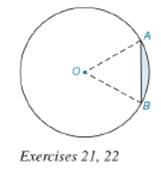Chapter 8.5, Problem 21E### Elementary Geometry for College St...

6th Edition
Daniel C. Alexander + 1 other
ISBN: 9781285195698

#### Solutions

Chapter
Section### Elementary Geometry for College St...

6th Edition
Daniel C. Alexander + 1 other
ISBN: 9781285195698
Textbook Problem
3 views

# Find the exact perimeter and area of the segment shown, given that m ∠ O = 60 0 and O A = 12 in.To determine

To find:

The exact perimeter and area of the shaded segment.

Explanation

Definition:

Arc Measure:

In a circle, the degree measure of an arc is equal to the measure of central angle that intercepts the arc.

Formula:

Perimeter of segment:

The perimeter of a segment is the sum lengths of chord and its associated arc.

Psegment= Length of chord + Length of associated arc.

Length of an arc:

In a circle whose circumference is C, the length l of an arc whose degree measure is m is given by:

l=m360×C

We know that, circumference of a circle is given by 2πr where r is the radius of circle.

Hence, length l of an arc =m360×2πr

Area of segment:

Asegment=Asector-P

Area of sector:

If r is the radius of the circle, the area A of a sector whose arc has degree measure m is given by

A=m360×πr2

Area of triangle:

If a, b and c are lengths of sides of triangle, then area of triangle is given by the formula:

A=s(s-a)(s-b)(s-c)

Where s is the semi perimeter which is given by s=12(a+b+c)

Calculation:

Perimeter of segment:

OA is the radius of circle. Thus, r=12 in

The measure of central angle is mO=600.

As per the definition of measure of an arc, the degree measure of an arc is equal to the central angle intercepted by it.

Thus, the degree measure of arc AB^ =600. Hence, m=600

Length l of an arc is given by the formula m360×2πr

Let’s substitute the value of m and r to find the length of arc.

Thus, the length of arc = 60360×2π×12

Simplify: l(AB^)=4π in.

In OAB, OA and OB are radii of the circle. Radii of circle are of equal length.

If two sides of a triangle are equal, angles opposite to equal sides are also equal.

Thus, OAB=OBA

In a triangle, sum of all angles = 1800

mO+mOAB+mOBA=1800

600+mOAB+mOBA=1800

mOAB+mOBA=1800-600

mOAB+mOBA=1200

Since both the angles are equal and their sum is 1200, each angle measures 600

### Still sussing out bartleby?

Check out a sample textbook solution.

See a sample solution

#### The Solution to Your Study Problems

Bartleby provides explanations to thousands of textbook problems written by our experts, many with advanced degrees!

Get Started

#### Find the limit: limx2x38x2.

Calculus: An Applied Approach (MindTap Course List)

#### Simplify: 151

Elementary Technical Mathematics

#### [(13)2]3

Applied Calculus for the Managerial, Life, and Social Sciences: A Brief Approach

#### Evaluate the indefinite integral. ex1+exdx

Single Variable Calculus: Early Transcendentals, Volume I